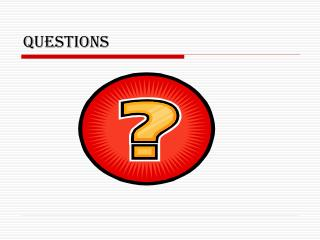DownloadDownload PresentationQUESTIONS

# QUESTIONS

Télécharger la présentation## QUESTIONS

- - - - - - - - - - - - - - - - - - - - - - - - - - - E N D - - - - - - - - - - - - - - - - - - - - - - - - - - -
##### Presentation Transcript

1. QUESTIONS

2. Homework • Jack load 12.2kN • Gage pressure: 27.7kPa

3. SG = 0.8

4. Examples • Mercury manometer is connected to an air duct to measure its insice pressure. The manometer deflection is 15mm. Atmospheric pressure is 100kPa. Find the duct’s absolute pressure. Hg  = 13,600kg/m3.

5. Manometer • Liquid flow deflection large; manometer fluid can be Hg, SG = 13.6 • Gas flow deflection small; manometer fluid SG <~ 0.9. Use inclined manometer

6. Example • Mott 3.71 Figure 3.36 Gage fluid has a SG = 0.87 and L = 115mm.  = 15o. Find PA.

7. Fluid statics • Liquid at rest: F = 0 • Horizontal: equal in all directions • Horizontal: dependent on depth • Vertical: pressure prism • P = h

8. Hydrostatic forces • Pressure prism • Distributed forces • Force → vector • Direction • Magnitude • Line of action: center of pressure

9. Fluid statics • F = hcA magnitude • hcp = hc + (I/ hcA) center of pressure

10. Submerged surface location • Top edge at liquid surface • Top edge submerged • Pressurized surface –equivalent depth of fluid • Inclined surface • Curved surface

11. Example • A heavy car veers off the road into a lake. It settles upright on the bottom. The front car door is 1.2m high and 1m wide. The top of the door is 8m below the lake surface. What is the force holding the door closed? Can the driver open the door? What can he do to survive?

12. Example • A rectangular gate is installed in a vertical wall of a reservoir. Find the magnitude of the resultant force and the center of pressure. What is the force of each latch?

13. Example • The square gate is eccentrically pivoted so that it automatically opens at a certain value of h. What is that value in terms of l ?

14. Homework • A gate for the spillway holds water at a normal high level mark. The spillway crest elevation is 2090.0 ft. The gate span is 30 ft. What is the force on the two pins holding the gate in place?

15. Homework • The air above the liquid is at 40psig. The SG of the liquid is 0.8. The rectangular gate is 1.0m wide; y1 = 1.0m; and y2 = 3m. What force P is needed to hold gate in place?

16. Homework • The inclined wall is 4m wide. Find the magnitude of the resultant force and the center of pressure.

17. Centroid of compound area • Moment of compound area about any axis is equal to sum of the moments of its parts around the same axis • = (A1 x1 + A2 x2)/ AT

18. Assignment • Mott Chapters 3 & 4

19. References • Images & examples • Fluid Mechanics Fundamentals & Applications, 6th Edition, Cengel & Cimbala, McGraw Hill • Applied Fluid Mechanics, 6th Edition, Mott, Prentice Hall • Engineering Fluid Mechanics, 5th Edition Crowe, & Roberson, Wiley • Which of the examples were helpful? • Why?